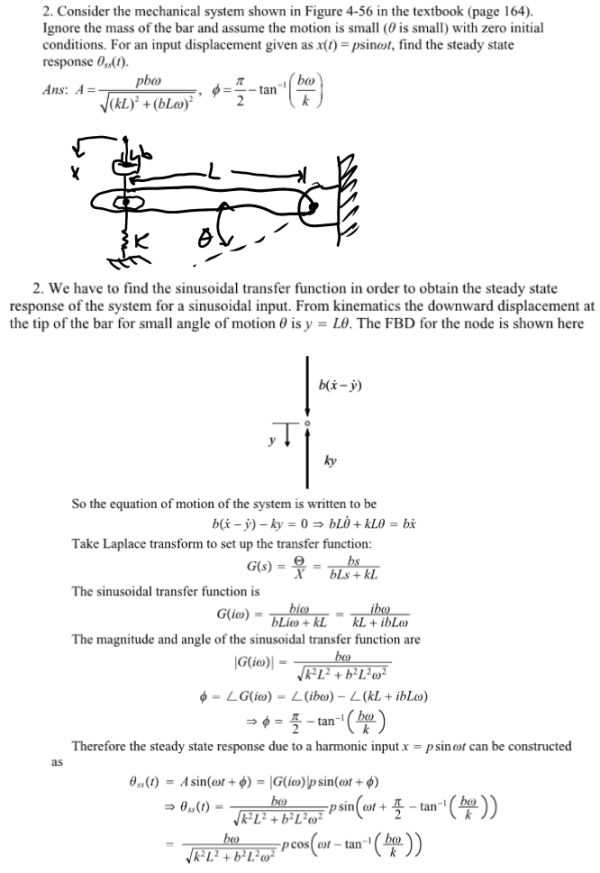consider the mechanical system shown in the figure in the textbook. Ignore the mass of the bar and assume the motion is small (theta is small) with zero initial conditions. For an input displacement given as x(t)=psinwt, find the steady state response.consider the mechanical system shown in the figure in the textbook. Ignore the mass of the bar and assume the motion is small (theta is small) with zero initial conditions. For an input displacement given as x(t)=psinwt, find the steady state response.

System Dynamics Page 2 dynamics dynamics dynamics dynamics dynamics dynamics dynamics System dynamics Page 3